# Math in Nature: 15 Stunning Ways We See Math in the World

## “Mathematics, rightly viewed, possesses not only truth but supreme beauty — a beauty cold and austere, without the gorgeous trappings of painting or music.” — Bertrand Russell.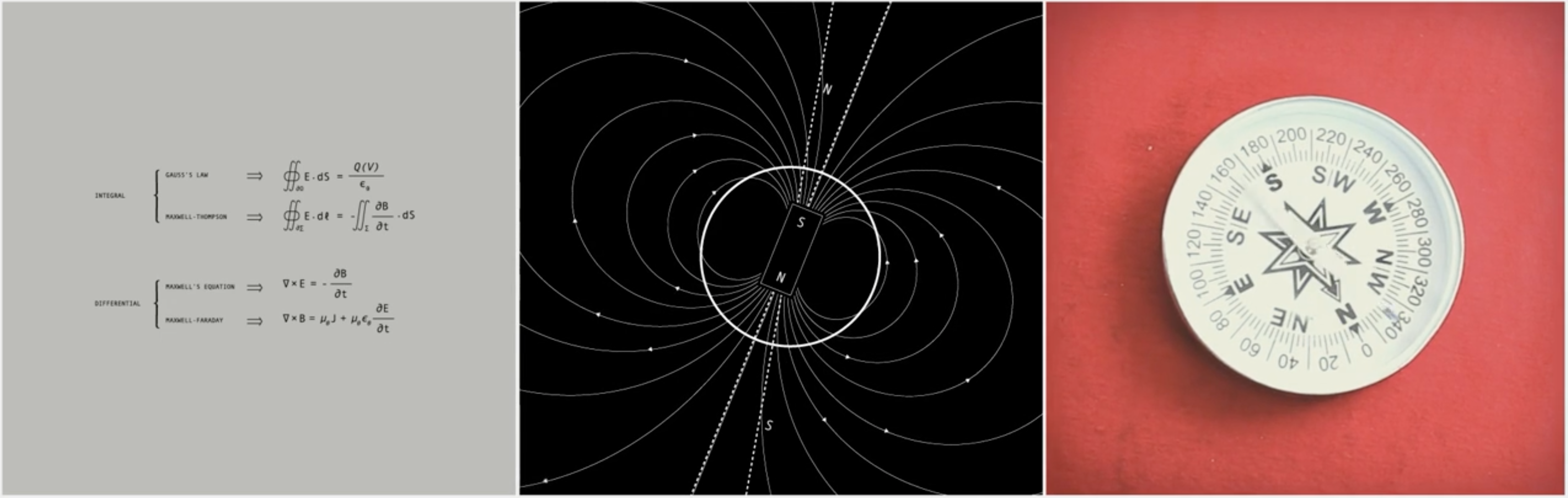Note: As an Amazon Associate I earn from qualifying purchases.

## The Mathematics Behind Light Waves

We use mathematics to get the simple harmonic motion of light waves, which are the neatest form of oscillations. Then we turn on the light.To understand the mathematics behind light waves, you can read this book.

## The Mathematical Theory Behind Backgammon

We use the conditional probability theory to see how likely it is that you’ll roll a two and a four during a game of backgammon.To understand the mathematical theory behind backgammon, you can read this book.

## The Mathematics Behind Snowflakes

No two snowflakes are alike. They are also beautiful fractals. The Koch snowflake is one of the earliest fractals to have been described. It demonstrates infinity, one of the most intriguing concepts of math. It has an infinite perimeter but a finite area.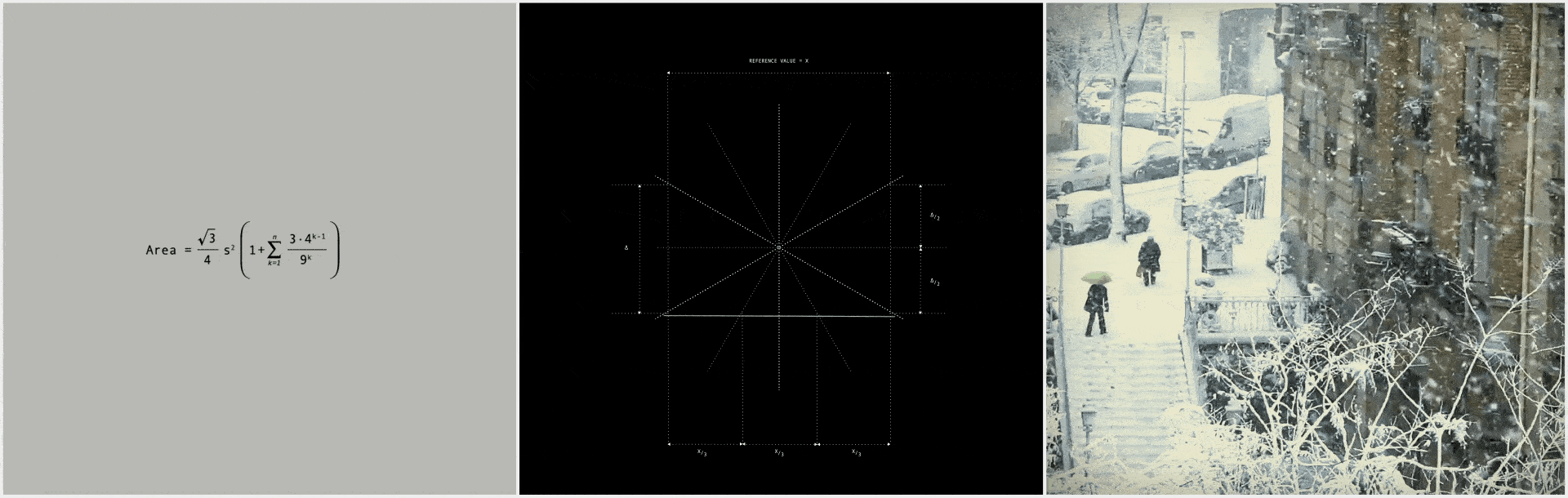To understand the mathematics behind snowflakes, you can read this book.

## The Mathematics of a Spinning Top

We use the equation of motion to model how fast a spinning top needs to go and how the topping point goes for when it wobbles out of control.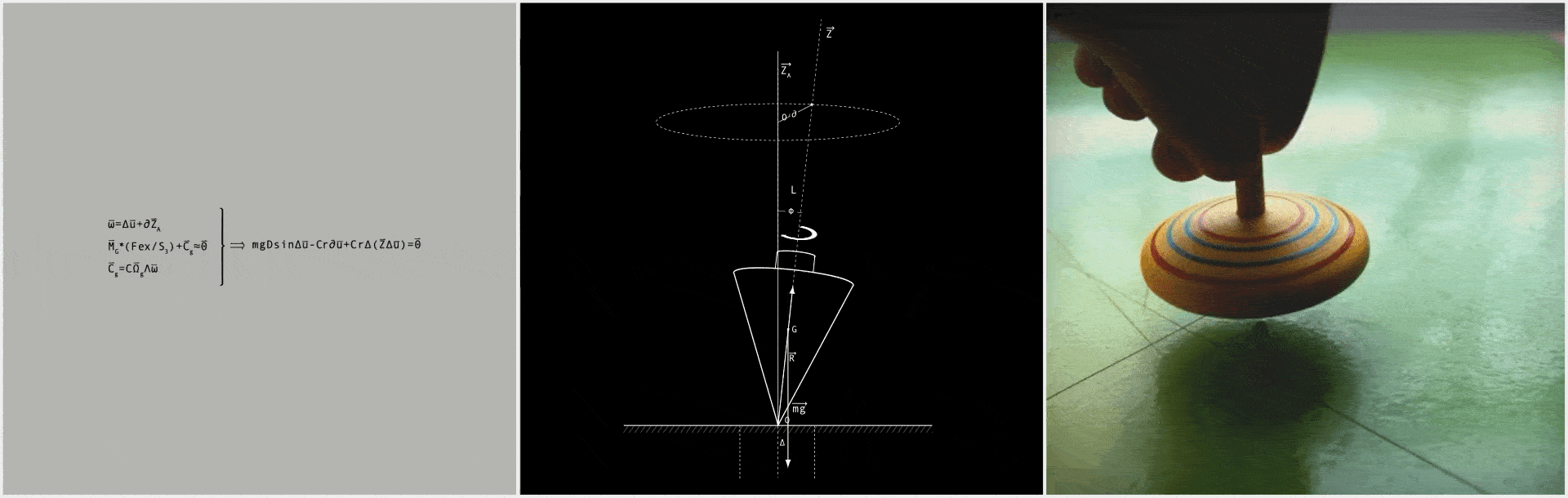To understand the mathematics behind a spinning top, you can read this book.

## The Mathematics Behind a Magnifying Glass

Optical spherical aberration is a property of optical systems such as lenses that causes light to be spread out over some space region rather than focused to a point. We use mathematics to calculate how a given size and proportion lens will magnify something at different distances. Then bespectacled people can see.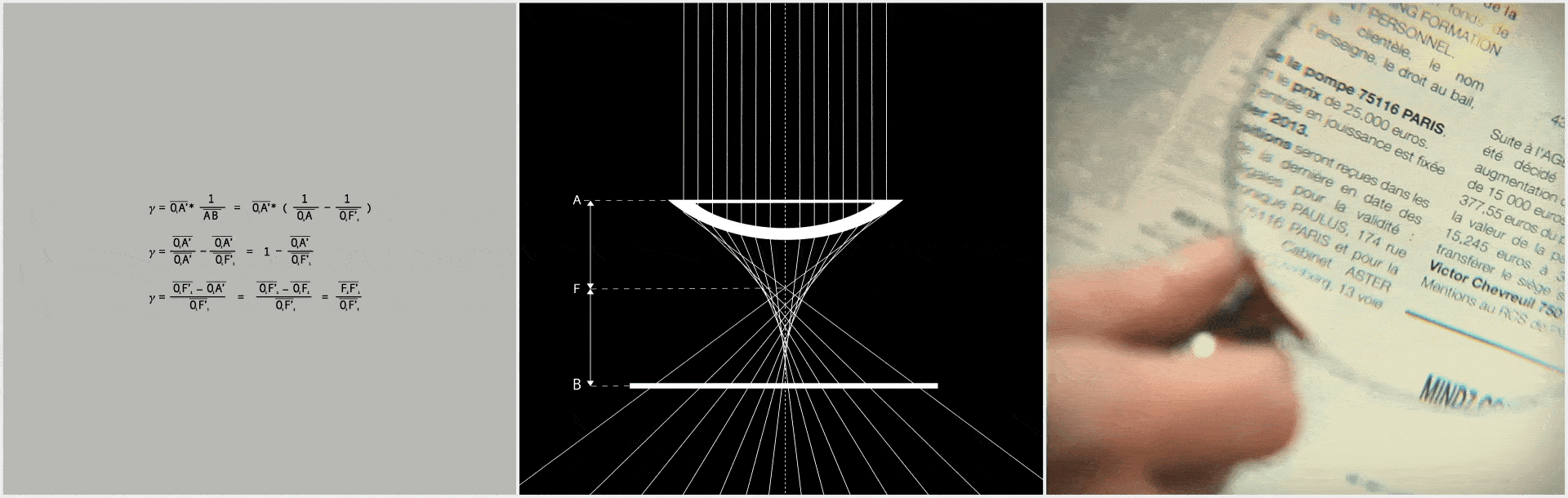To understand the mathematics behind a magnifying glass, you can read this book.

## The Mathematics Behind a Compass

Maxwell’s equations are coupled partial differential equations that form the foundation of classical electromagnetism, classical optics, and electric circuits. They help us describe how electromagnetism and compass work. Then we don’t get lost.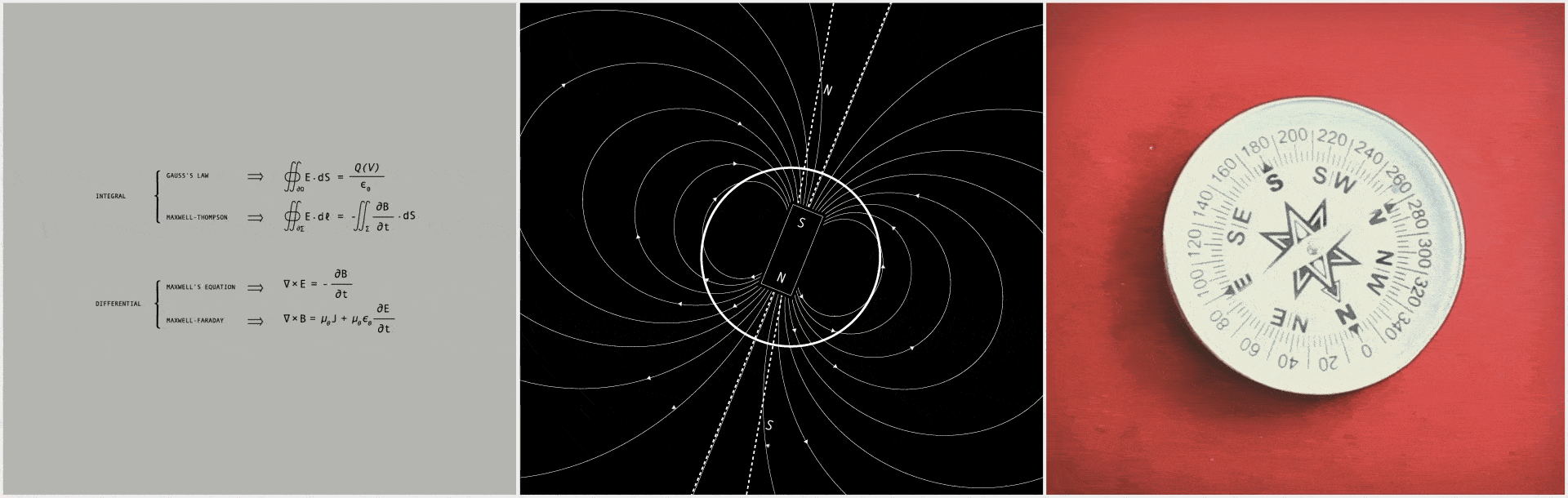To understand the mathematics behind a compass, you can read this book.

## The Mathematics Behind a Cup of Coffee

In chemistry, the molar mass is utterly essential when setting up an experiment. It allows you to figure out how much you should weigh out substances on your scale. Then we can figure out how much sugar we need to add to our coffee to stay healthy.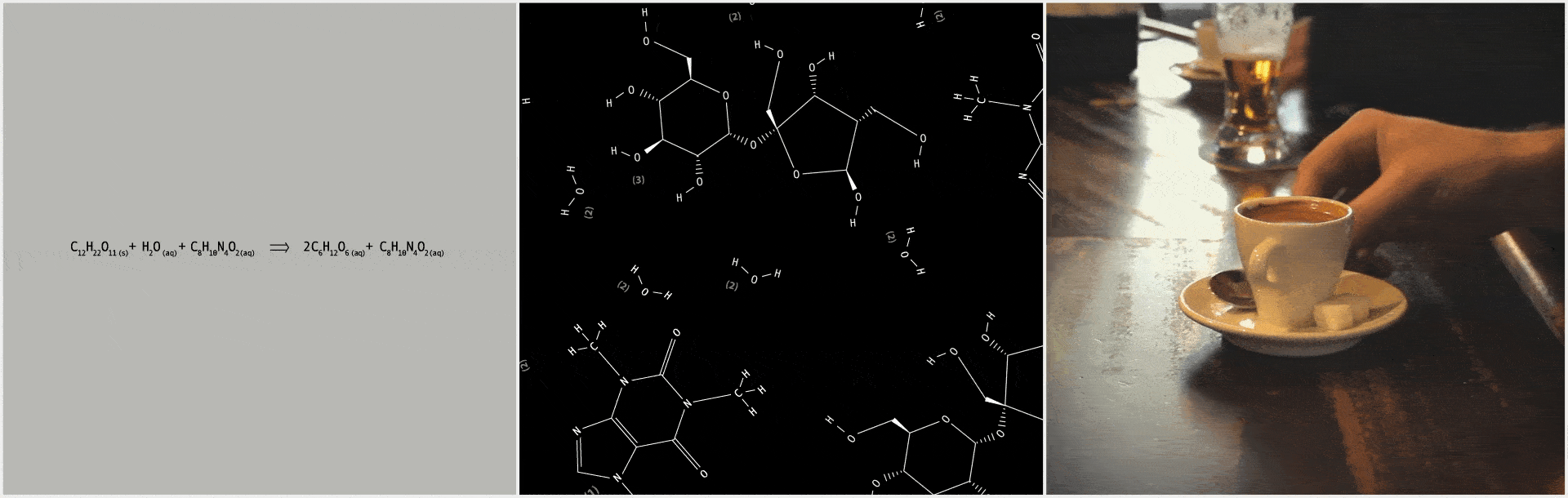To understand the mathematics behind a cup of coffee, you can read this book.

## The Mathematics Behind Weather Forecasting

Numerical weather prediction (NWP) uses the atmosphere and oceans’ mathematical models to predict the weather based on current weather conditions. Then we decide on the clothes we will wear today.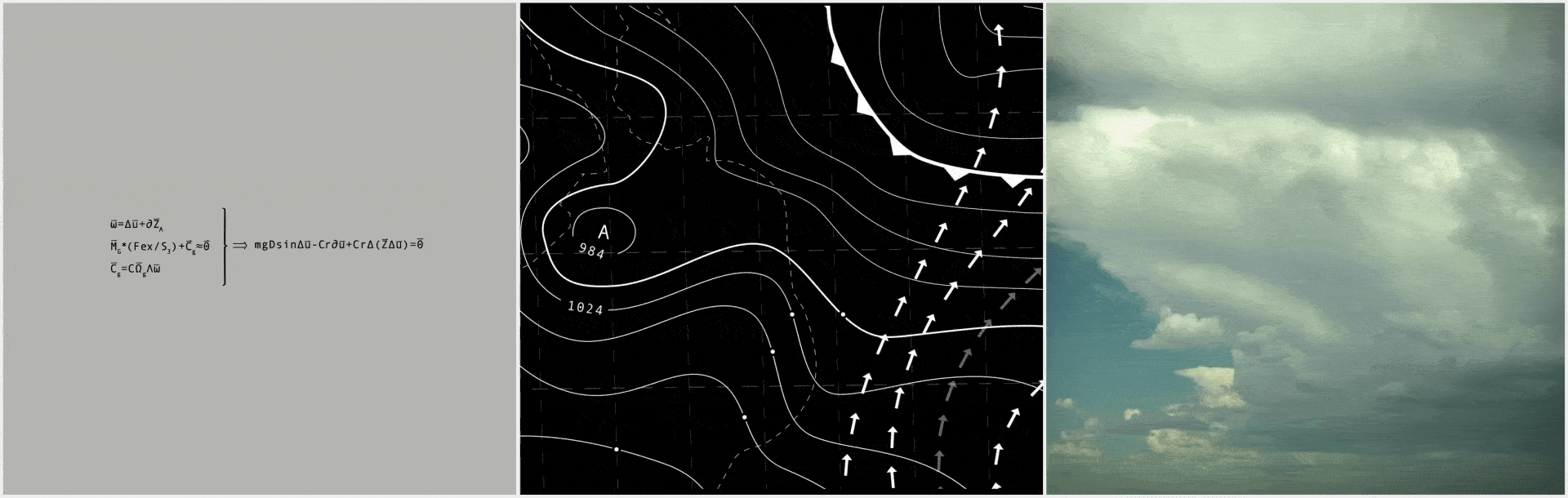To understand the mathematics behind weather forecasting, you can read this book.

## The Mathematics Behind a Computer Screen

In mathematics and digital electronics, a binary number is expressed in the base-2 numeral system or binary numeral system, which uses only two symbols: typically 0 and 1. Math is the reason a series of ones and zeros can end up on your screen as what you’re reading right now. Then we can read this post.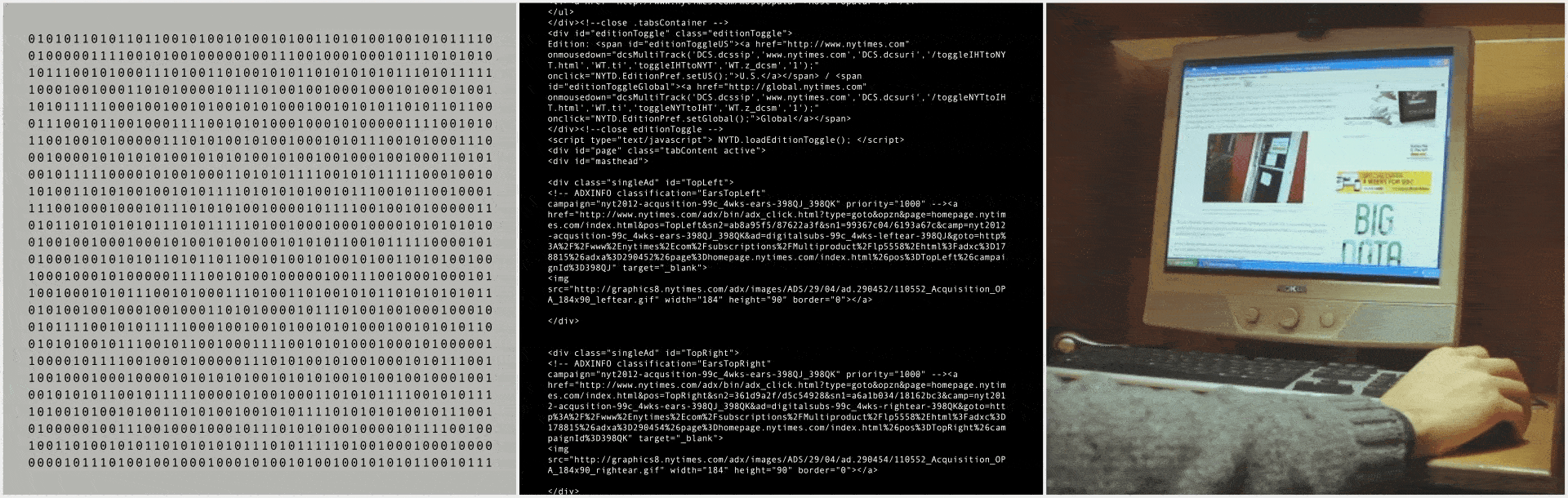To understand the mathematics behind a computer screen, you can read this book.

## The Mathematics Behind Tree Growing

The Mandelbrot set is the set of complex numbers for which the function does not diverge when iterated from remains bounded in absolute value. A binary fractal tree is defined recursively by symmetric binary branching.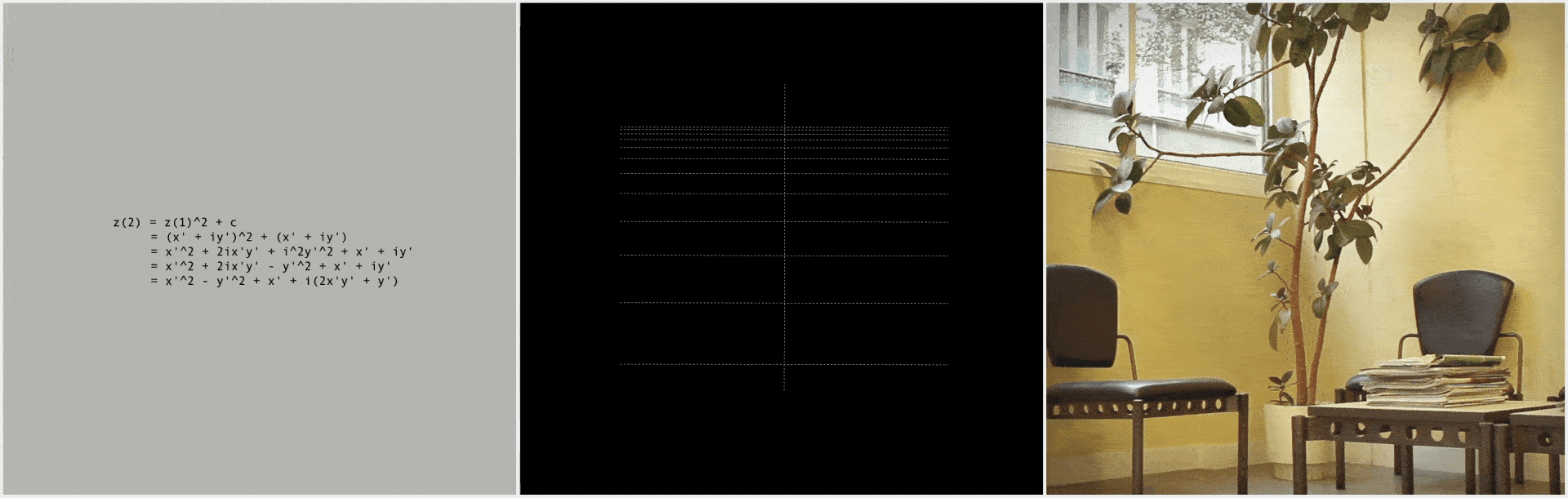To understand the mathematics behind tree growing, you can read this book.

## The Mathematics Behind Human Genetic & Fingerprinting

Mathematics makes possible the management and analysis of the massive database of the Human Genome. The importance of mathematics and statistics in genetics is well known. A genetic algebra is an algebra used to model inheritance in genetics.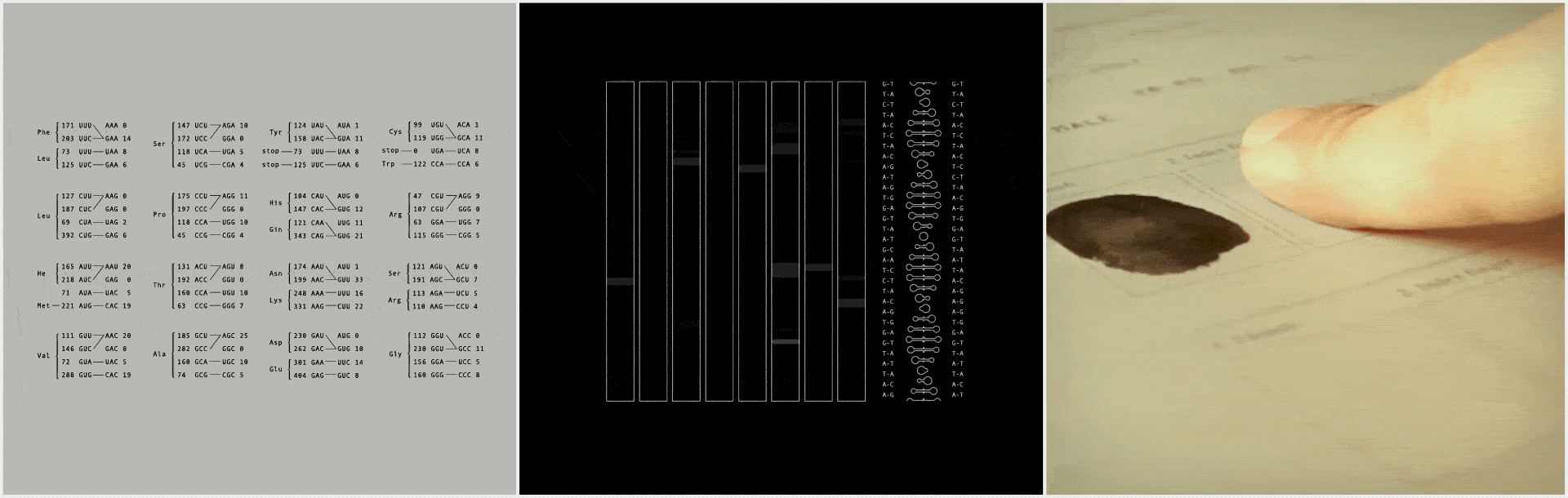To understand the mathematics behind human genetic and fingerprinting, you can read this book.

## The Mathematics Behind Music & Sound

The Z-Transform allows us to analyze a system’s sinusoidal components’ frequency and phase to characterize a system’s response. It makes digital signal processing possible. Then we can listen to Brazzaville while we are walking in Manhattan.To understand the mathematics behind music and sound, you can read this book.

## The Mathematics Behind Fuel Prices

We do the maths on how petrol prices are calculated and show that one element is rising fast, masked by the falling crude oil cost. Then we can make the economy safe.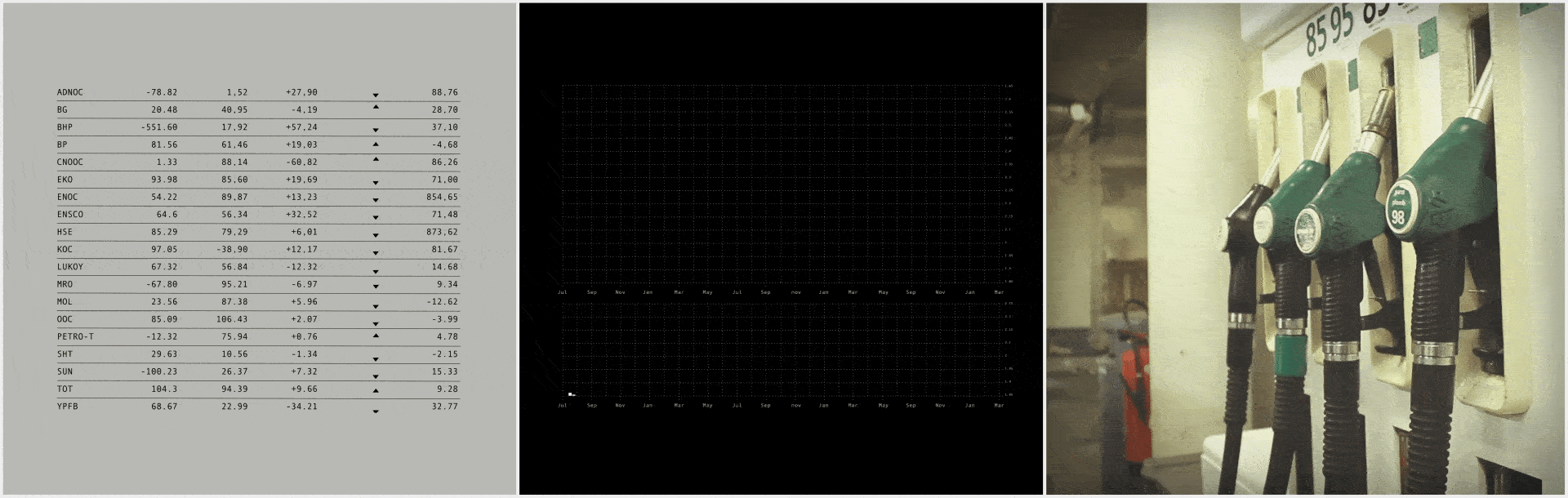To understand the mathematics behind fuel prices, you can read this book.

## The Mathematics Behind Airplane Takeoff

A lift coefficient is a number that aerodynamicists use to model all of the complex dependencies of shape, inclination, and flow conditions on a lift. That makes a flight possible. Then we can go to Argentina whenever we want.To understand the mathematics behind airplane takeoff, you can read this book.

## The Mathematics Behind Force-Sensing Resistor

A force-sensing resistor is a material whose resistance changes when a force, pressure, or mechanical stress is applied. It makes our modems work correctly and let us stay connected.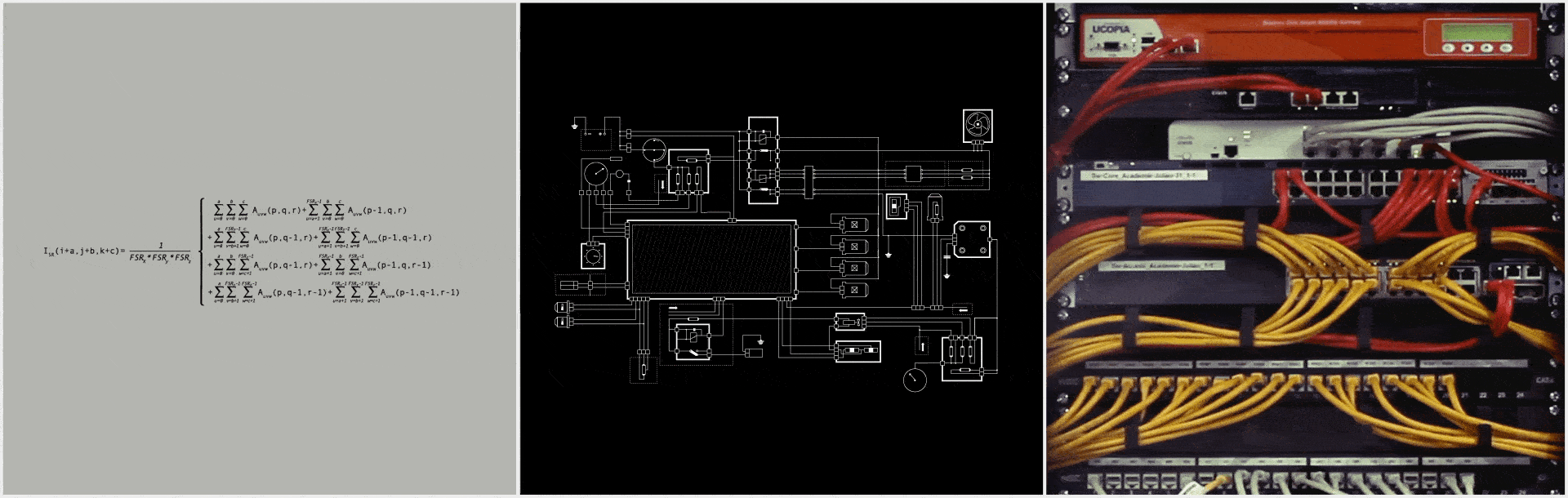To understand the mathematics behind force-sensing resistor, you can read this book.
`Note: As an Amazon Associate I earn from qualifying purchases.`

I found tons of books in Argentina!